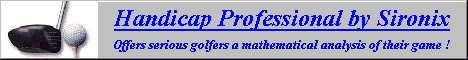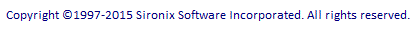Rate of Return Calculator The interactive calculator below demonstrates some very simple performance calculations. Enter a start date and value, up to 5 intervening transactions, and an end date and value; then click the Calculate Results button. It will calculate dollar gain, simple roi %, and annualized compound rate of return. (click here to review performance measurement basics)